### Cardinal Directions

The four cardinal directions or cardinal points are north, south, east, and west.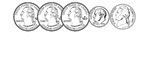### 90 Cents

Groups of change with totals from 1 to 100 cents using the least amount of coins.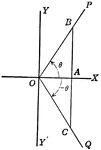### Coordinate Axis With Perpendiculars Drawn To Form Similar Right Triangles From Positive and Negative Theta, Θ

Angle XOP=Θ and angle XOQ=- Θ. From a point in the terminal side of each a perpendicular line is drawn…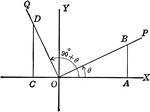### Coordinate Axis With Perpendiculars Drawn To Form Similar Right Triangles

Angle XOP=Θ and angle XOQ=90+Θ. From a point in the terminal side of each a perpendicular line is…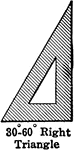### 30-60-90 Right Triangle

30-60-90 right triangle.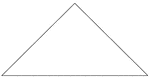### Isosceles Triangle degrees 90, 45, 45

An isosceles triangle with angles 90, 45, 45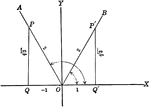### Trigonometric Reference Triangles/Angles (60 degrees) Drawn in Quadrants

Trigonometric reference triangles/angles drawn for 60 degree reference angel in quadrants I and II.### Right Triangle OCB With, x, y, and r shown

Right triangle OCB that can be used to show the relationships between x, y, r, and Θ.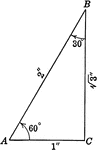### Special Right Triangle with Angles 30, 60, 90 degrees

Special right triangle with angles 30 degrees, 60 degrees, and 90 degrees with side measures/relationships…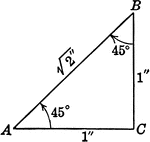### Special Right Triangle with Angles 45, 45, 90 degrees

Special right triangle with angles 45 degrees, 45 degrees, and 90 degrees with side measures/relationships…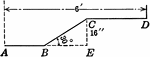### Triangle Steam Pipe

Steam pipe illustration of 30-60-90 triangle.